# What is Phase,Phase Angle,Phase Difference Easy Understanding

## What is Phase:

The phase is defined as the position of the waveform at a fraction of time period. Phase is expressed in angle or radian. Phase can also be an expression of relative displacement between two corresponding features (for example, peaks or zero crossings) of two waveforms having the same frequency.

Note: one radian of phase equals approximately 57.3°. Consider the sinusoidal wave in the diagram. Which has complete cycle, the phase of the current at point A is to be found. If ‘T’ is the time period, note that is this point the time is T/4. Here the phase at that given time is pi/2. Let get through expression….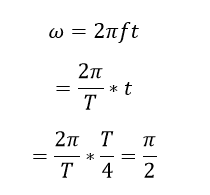## Phase difference:

Phase difference is the difference, between two waves is having the same frequency and referenced to the same point in time. It is expressed in degrees or radians. Let’s consider two sinusoidal wave, both have same frequency, Example: R phase and B phase (in our three-phase circuit.)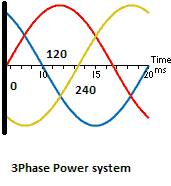Here both the wave is having 50 Hz frequency, and R phase starts from 0 deg and B phase starts from 120 deg from R phase of the electrical circuit. Hence the phase difference isNote: Consider R phase as a reference phase.

Generally

– symbol indicates leading nature (the wave leads the angle from the reference wave)

+ symbol indicates lagging nature (the wave lags the angle from the reference wave)

## Phase angle:

In the context of phasors, phase angle refers to the angular component of the complex number representation of the function. The notation of the phase angle is defined as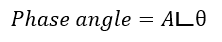A is the magnitude and phase angle θ, is called angle notation. This notation is mostly used in electrical circuit to represent an electrical impedance (vector sum of resistance and reactance) and the apparent power (vector sum of real power and reactive power). Here the phase angle theta is the phase difference between the voltage applied to the impedance and the current flow through the impedance.

In the context of periodic phenomena, such as a wave, phase angle is synonymous with phase.

## Bioelectric impedance

In bioelectrical impedance analysis in humans, an estimate of the phase angle can be obtained and is based on changes in resistance and reactance as alternating current passes through tissues, which causes a phase shift. The measured phase angle therefore depends on several biological factors. Phase angle is greater in men than women, and decreases with increasing age

## In phase:

The two waveform said to be in phase where the two wave should reach maximum, minimum and zero values simultaneously at the same time.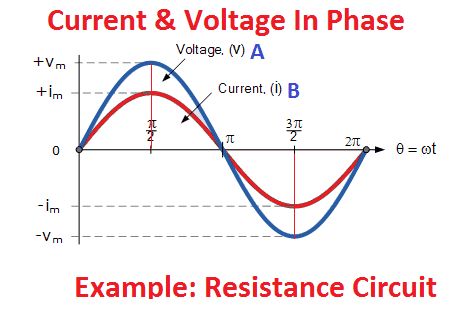Example: Heater Circuit

Check the Waveform:

The two sine wave both reaches maximum value at point A, zero at point B and minimum at point C.

## Out Phase:

Two sinusoidal signals are said to be out of phase when they do not reach maximum or zero values at the same time.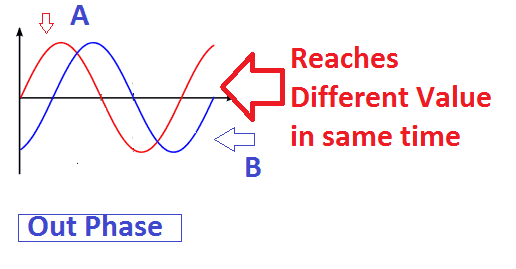Example: three-phase voltage

Check the Waveform:

Here the diagram, both the wave reaches different values at same time.

#### 1 COMMENT

1. Hi, the What is Phase,Phase Angle,Phase Difference Easy Understanding is very good, congratulations to electrical4u.net’ authors.